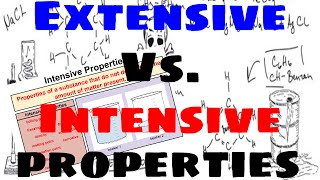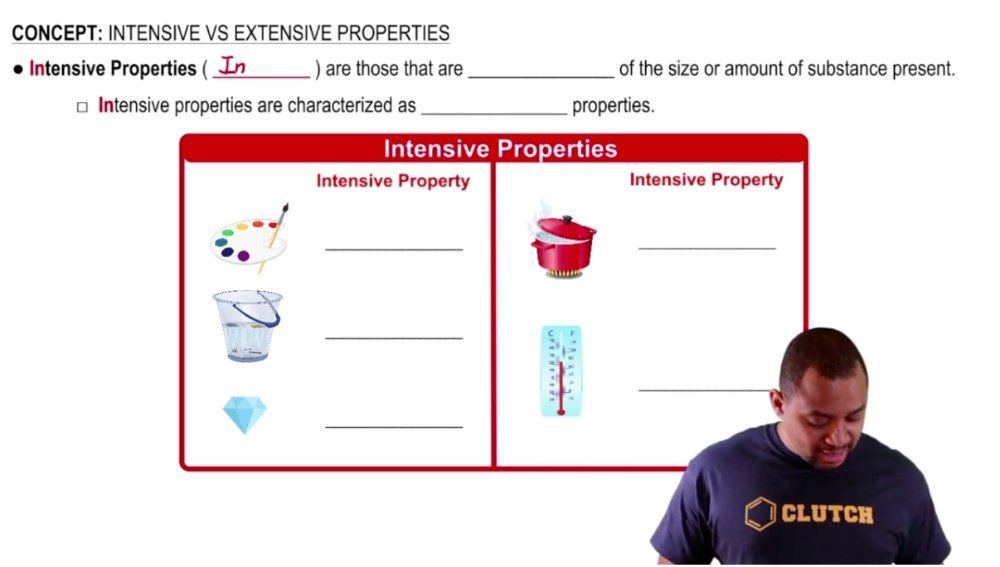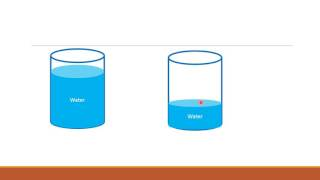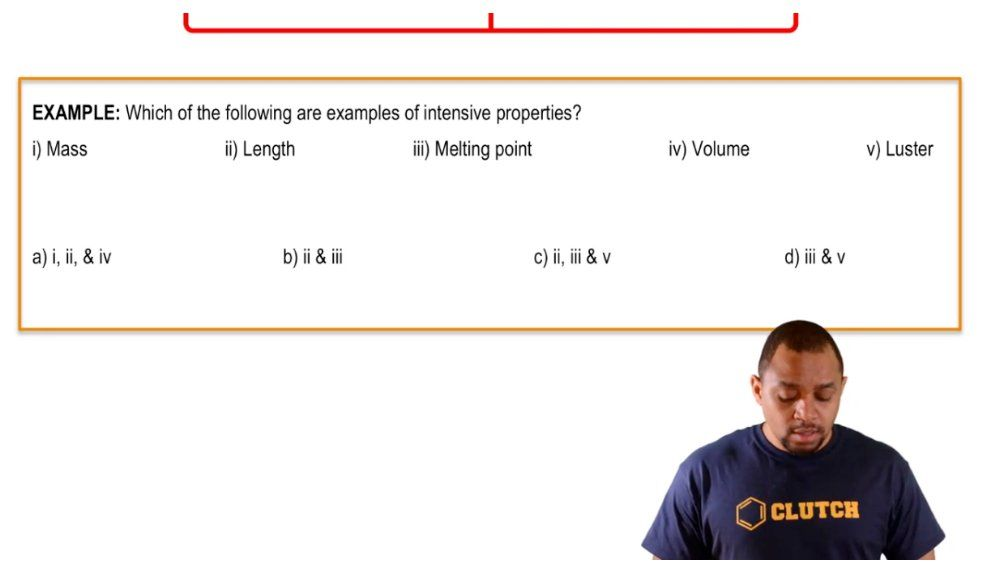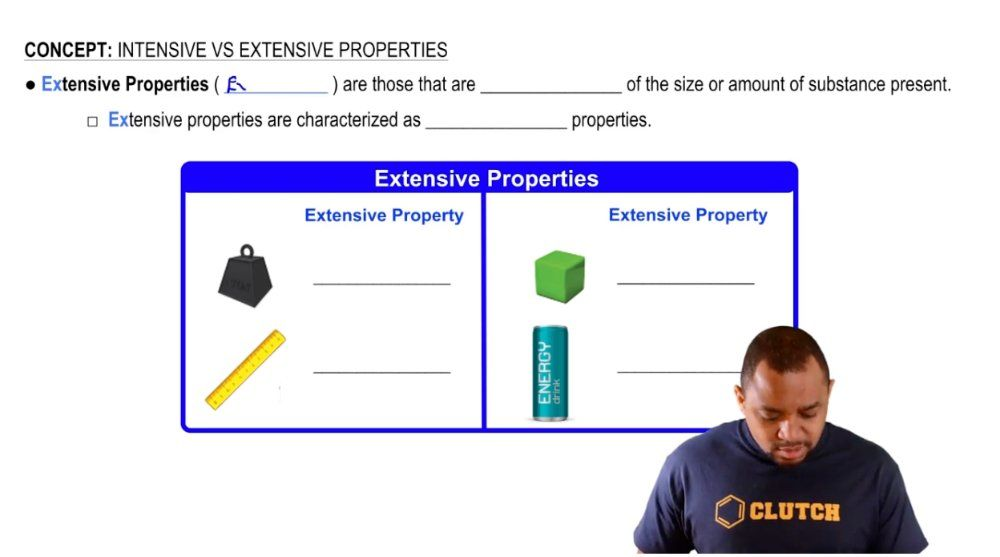Start typing, then use the up and down arrows to select an option from the list.
1. 1. Intro to General Chemistry2. Intensive vs. Extensive Properties
Problem

# Label the following statements about the world’s largest gold bar as quantitative or qualitative observations. (This gold bar was worth approximately \$10.25 million in 2013.) (a) The melting point of gold is 1064.2 °C. (b) The volume of the gold bar is 15,730 cm3. (c) Gold metal is a conductor of electricity. (d) The mass of the gold bar is 250 kg. (e) The gold bar is yellow and shiny

Relevant Solution2m
Play a video:
Hello everyone today. We are being given the following problem, classify the following properties of zinc as quantitative or qualitative. First you wanted to find what each of these mean. So when something is quantitative, this means that it can be measured with a numerical value. So whenever you measure anything within the numerical value it's going to be quantitative tells you how much on the other hand, qualitative. So qualitative on the other hand are things that can be observed but not measured with numerical values so it can only be observed but not measured with numbers. And so we can therefore answer our questions now. So far. First part says Zinc melts at 419.5°C. This is going to be quantitative and I'll just put quan for short quantitative. For our second part zinc has a bluish silver color. This isn't a numerical value, it's an observation. And so too is going to be qualitative or qualify for short For three. The density of zinc is 7.14 g per centimeter cubed. There is a numerical value that has been measured. So this is therefore going to be quantitative. And for the fourth one at room temperature, zinc is brittle and so there's no numerical value. There's just an observation. So that's gonna be quantitative. And so our correct answer is going to be answer choice B Statements one in are quantitative While statements two and 4 are qualitative and with that we've answered the question overall. I hope this helped. And until next time